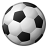Announcements
[WEBINAR] Accenture & Qlik: Accelerating BI Migration to SaaS with Qlik on Dec 13th: REGISTER
cancel
Showing results for
Did you mean:Creator

## Slider variables not linking correctly with rolling 12 months

Morning all, qlikview fun ever increasing and I hope you can help with my latest queryAs per my attached, I am trying to create a rolling 12 months calculation, that works with a slider calculation, based on min and max variables.

I have 3 straight tables, showing how the example should work.

1. what the total amount should be
2. rolling 12 month calculation
3. rolling 12 month calculation, which includes the slider min and max variables.

So when you click period 1217, the rolling 12 months amount stays the same which is excellent.

However the final straight table, including the slider variables decreases in amount.

Any advice/help as to what i'm doing wrong would be fantastic?

I'm hoping it's just a missing bracket or some sort, which I just cannot see (it's weird, as it works with the year, just not the period).

Much appreciated.

1 Solution

Accepted SolutionsMVP

I think you need to add the same date and time set analysis to your condition on Client

=Sum({<[Period_Sort]={">=\$(=max([Period_Sort])-11)<=\$(=max([Period_Sort]))"},Period_Number= ,Year_YYYY= ,

Client_Name={"=round(Sum({<[Period_Sort]={"">=\$(=max([Period_Sort])-11)<=\$(=max([Period_Sort]))""},Period_Number= ,Year_YYYY=>}Revenue))<=\$(vMinLimit) or round(Sum({<[Period_Sort]={"">=\$(=max([Period_Sort])-11)<=\$(=max([Period_Sort]))""},Period_Number= ,Year_YYYY=>}Revenue))>=\$(vMaxLimit)"}>} Revenue)3 RepliesMVP

I think you need to add the same date and time set analysis to your condition on Client

=Sum({<[Period_Sort]={">=\$(=max([Period_Sort])-11)<=\$(=max([Period_Sort]))"},Period_Number= ,Year_YYYY= ,

Client_Name={"=round(Sum({<[Period_Sort]={"">=\$(=max([Period_Sort])-11)<=\$(=max([Period_Sort]))""},Period_Number= ,Year_YYYY=>}Revenue))<=\$(vMinLimit) or round(Sum({<[Period_Sort]={"">=\$(=max([Period_Sort])-11)<=\$(=max([Period_Sort]))""},Period_Number= ,Year_YYYY=>}Revenue))>=\$(vMaxLimit)"}>} Revenue)Creator
Author

sunny you absolute legend.

makes complete sense, to include on the client side too and im pleased to report, this works like a dream.

you've started my weekend off in great style.

I hope you have an awesome fridayMVP

Thanks Marcus, you have a good rest of the weekend my friendCommunity Browser